# 二、端到端的机器学习项目

1. 项目概述。
2. 获取数据。
3. 发现并可视化数据，发现规律。
4. 为机器学习算法准备数据。
5. 选择模型，进行训练。
6. 微调模型。
7. 给出解决方案。
8. 部署、监控、维护系统。

## 使用真实数据

• 流行的开源数据仓库：
• 准入口（提供开源数据列表）
• 其它列出流行开源数据仓库的网页：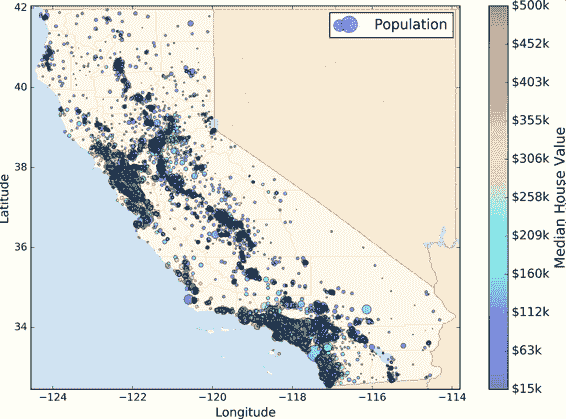## 项目概览

### 划定问题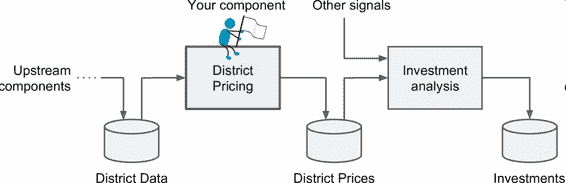OK，有了这些信息，你就可以开始设计系统了。首先，你需要划定问题：监督或非监督，还是强化学习？这是个分类任务、回归任务，还是其它的？要使用批量学习还是线上学习？继续阅读之前，请暂停一下，尝试自己回答下这些问题。

### 选择性能指标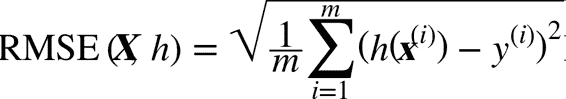• m是测量 RMSE 的数据集中的实例数量。
例如，如果用一个含有 2000 个街区的验证集求 RMSE，则m = 2000

• x^(i)是数据集第i个实例的所有特征值（不包含标签）的向量，y^(i)是它的标签（这个实例的输出值）。

例如，如果数据集中的第一个街区位于经度 –118.29°，纬度 33.91°，有 1416 名居民，收入中位数是 38372 美元，房价中位数是 156400 美元（忽略掉其它的特征），则有：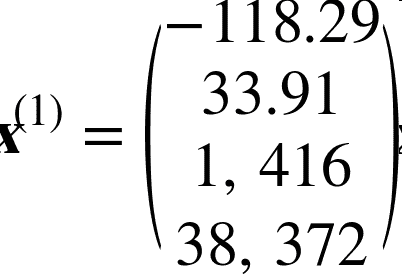和，• X是包含数据集中所有实例的所有特征值（不包含标签）的矩阵。每一行是一个实例，第i行是x^(i)的转置，记为x^(i)^T

![](img/o-2-3.png)

• h是系统的预测函数，也称为假设（hypothesis）。当系统收到一个实例的特征向量x^(i)，就会输出这个实例的一个预测值y_hat = h(x^(i))y_hat读作y-hat）。

例如，如果系统预测第一区的房价中位数是 158400 美元，则y_hat^(1) = h(x^(1)) = 158400。预测误差是 y_hat^(1) - y^(1) = 2000

• RMSE(X,h)是使用假设h在样本集上测量的损失函数。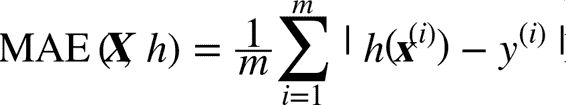RMSE 和 MAE 都是测量预测值和目标值两个向量距离的方法。有多种测量距离的方法，或范数：

• 计算对应欧几里得范数的平方和的根（RMSE）：这个距离介绍过。它也称作ℓ2范数，标记为||·||₂（或只是||·||）。

• 计算对应于ℓ1（标记为||·||₁）范数的绝对值和（MAE）。有时，也称其为曼哈顿范数，因为它测量了城市中的两点，沿着矩形的边行走的距离。

• 更一般的，包含n个元素的向量vℓk范数（K 阶闵氏范数），定义成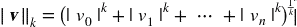ℓ0（汉明范数）只显示了这个向量的基数（即，非零元素的个数），ℓ∞（切比雪夫范数）是向量中最大的绝对值。

• 范数的指数越高，就越关注大的值而忽略小的值。这就是为什么 RMSE 比 MAE 对异常值更敏感。但是当异常值是指数分布的（类似正态曲线），RMSE 就会表现很好。

## 获取数据

### 创建工作空间

pip 9.0.1 from [...]/lib/python3.5/site-packages (python 3.5)



$pip3 install --upgrade pip Collecting pip [...] Successfully installed pip-9.0.1  创建独立环境 如果你希望在一个独立环境中工作（强烈推荐这么做，不同项目的库的版本不会冲突），用下面的pip命令安装virtualenv $ pip3 install --user --upgrade virtualenv Collecting virtualenv [...] Successfully installed virtualenv




$cd$ML_PATH
$virtualenv env Using base prefix '[...]' New python executable in [...]/ml/env/bin/python3.5 Also creating executable in [...]/ml/env/bin/python Installing setuptools, pip, wheel...done.  以后每次想要激活这个环境,只需打开一个终端然后输入： $ cd $ML_PATH$ source env/bin/activate


$pip3 install --upgrade jupyter matplotlib numpy pandas scipy scikit-learn Collecting jupyter Downloading jupyter-1.0.0-py2.py3-none-any.whl Collecting matplotlib [...]  要检查安装，可以用下面的命令引入每个模块： $ python3 -c "import jupyter, matplotlib, numpy, pandas, scipy, sklearn"



\$ jupyter notebook
[I 15:24 NotebookApp] Serving notebooks from local directory: [...]/ml
[I 15:24 NotebookApp] 0 active kernels
[I 15:24 NotebookApp] The Jupyter Notebook is running at: http://localhost:8888/
[I 15:24 NotebookApp] Use Control-C to stop this server and shut down all
kernels (twice to skip confirmation).



Jupyter 服务器现在运行在终端上，监听 8888 端口。你可以用浏览器打开http://localhost:8888/，以访问这个服务器（服务器启动时，通常就自动打开了）。你可以看到一个空的工作空间目录（如果按照先前的virtualenv步骤，只包含env目录）。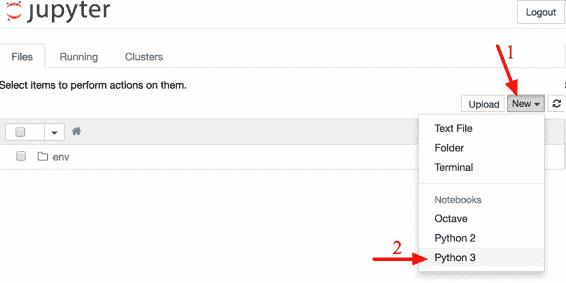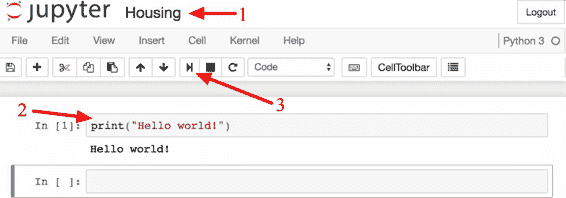### 下载数据

import os
import tarfile
from six.moves import urllib

HOUSING_PATH = "datasets/housing"

def fetch_housing_data(housing_url=HOUSING_URL, housing_path=HOUSING_PATH):
if not os.path.isdir(housing_path):
os.makedirs(housing_path)
tgz_path = os.path.join(housing_path, "housing.tgz")
urllib.request.urlretrieve(housing_url, tgz_path)
housing_tgz = tarfile.open(tgz_path)
housing_tgz.extractall(path=housing_path)
housing_tgz.close()



import pandas as pd

csv_path = os.path.join(housing_path, "housing.csv")



### 快速查看数据结构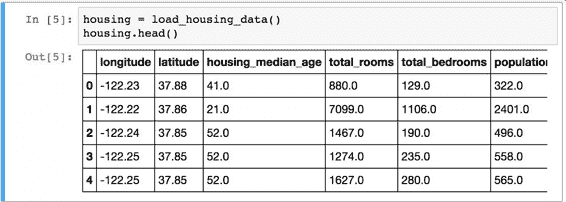info()方法可以快速查看数据的描述，特别是总行数、每个属性的类型和非空值的数量（见图 2-6）。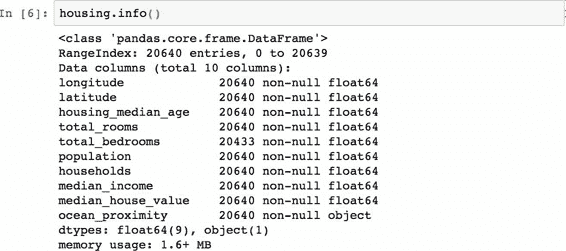>>> housing["ocean_proximity"].value_counts()
<1H OCEAN     9136
INLAND        6551
NEAR OCEAN    2658
NEAR BAY      2290
ISLAND           5
Name: ocean_proximity, dtype: int64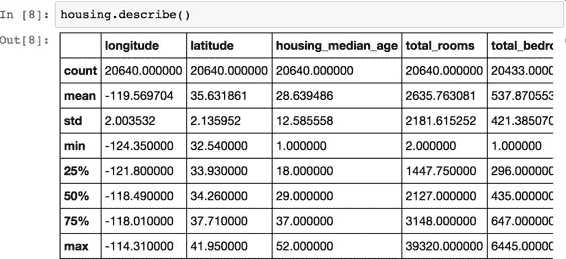countmeanminmax几行的意思很明显了。注意，空值被忽略了（所以，卧室总数是 20433 而不是 20640）。std是标准差（揭示数值的分散度）。25%、50%、75% 展示了对应的分位数：每个分位数指明小于这个值，且指定分组的百分比。例如，25% 的街区的房屋年龄中位数小于 18，而 50% 的小于 29，75% 的小于 37。这些值通常称为第 25 个百分位数（或第一个四分位数），中位数，第 75 个百分位数（第三个四分位数）。

%matplotlib inline   # only in a Jupyter notebook
import matplotlib.pyplot as plt
housing.hist(bins=50, figsize=(20,15))
plt.show()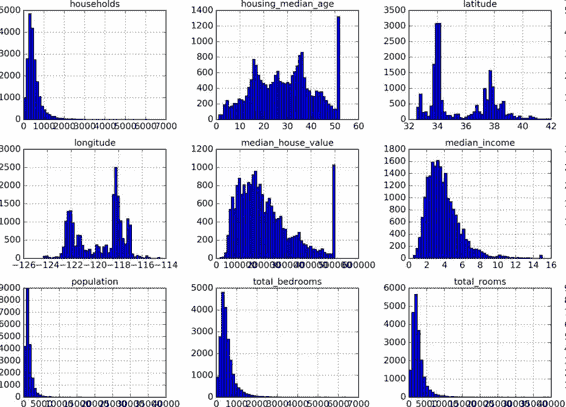1. 首先，收入中位数貌似不是美元（USD）。与数据采集团队交流之后，你被告知数据是经过缩放调整的，过高收入中位数的会变为 15（实际为 15.0001），过低的会变为 5（实际为 0.4999）。在机器学习中对数据进行预处理很正常，这不一定是个问题，但你要明白数据是如何计算出来的。

2. 房屋年龄中位数和房屋价值中位数也被设了上限。后者可能是个严重的问题，因为它是你的目标属性（你的标签）。你的机器学习算法可能学习到价格不会超出这个界限。你需要与下游团队核实，这是否会成为问题。如果他们告诉你他们需要明确的预测值，即使超过 500000 美元，你则有两个选项：

1. 对于设了上限的标签，重新收集合适的标签；
2. 将这些街区从训练集移除（也从测试集移除，因为若房价超出 500000 美元，你的系统就会被差评）。
3. 这些属性值有不同的量度。我们会在本章后面讨论特征缩放。

4. 最后，许多柱状图的尾巴很长：相较于左边，它们在中位数的右边延伸过远。对于某些机器学习算法，这会使检测规律变得更难些。我们会在后面尝试变换处理这些属性，使其变为正态分布。

### 创建测试集

import numpy as np

def split_train_test(data, test_ratio):
shuffled_indices = np.random.permutation(len(data))
test_set_size = int(len(data) * test_ratio)
test_indices = shuffled_indices[:test_set_size]
train_indices = shuffled_indices[test_set_size:]
return data.iloc[train_indices], data.iloc[test_indices]



>>> train_set, test_set = split_train_test(housing, 0.2)
>>> print(len(train_set), "train +", len(test_set), "test")
16512 train + 4128 test



import hashlib

def test_set_check(identifier, test_ratio, hash):
return hash(np.int64(identifier)).digest()[-1] < 256 * test_ratio

def split_train_test_by_id(data, test_ratio, id_column, hash=hashlib.md5):
ids = data[id_column]
in_test_set = ids.apply(lambda id_: test_set_check(id_, test_ratio, hash))
return data.loc[~in_test_set], data.loc[in_test_set]


housing_with_id = housing.reset_index()   # adds an index column
train_set, test_set = split_train_test_by_id(housing_with_id, 0.2, "index")


housing_with_id["id"] = housing["longitude"] * 1000 + housing["latitude"]
train_set, test_set = split_train_test_by_id(housing_with_id, 0.2, "id")


Scikit-Learn 提供了一些函数，可以用多种方式将数据集分割成多个子集。最简单的函数是train_test_split，它的作用和之前的函数split_train_test很像，并带有其它一些功能。首先，它有一个random_state参数，可以设定前面讲过的随机生成器种子；第二，你可以将种子传递给多个行数相同的数据集，可以在相同的索引上分割数据集（这个功能非常有用，比如你的标签值是放在另一个DataFrame里的）：

from sklearn.model_selection import train_test_split

train_set, test_set = train_test_split(housing, test_size=0.2, random_state=42)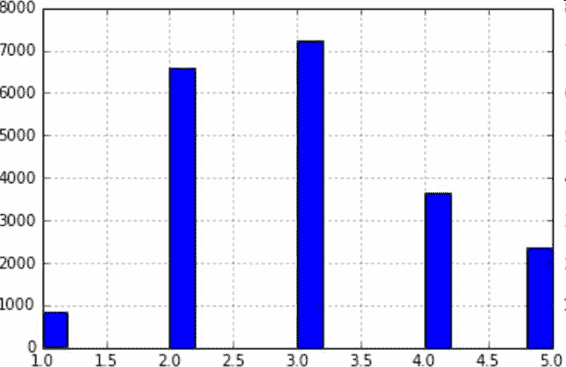housing["income_cat"] = np.ceil(housing["median_income"] / 1.5)
housing["income_cat"].where(housing["income_cat"] < 5, 5.0, inplace=True)


from sklearn.model_selection import StratifiedShuffleSplit

split = StratifiedShuffleSplit(n_splits=1, test_size=0.2, random_state=42)

for train_index, test_index in split.split(housing, housing["income_cat"]):
strat_train_set = housing.loc[train_index]
strat_test_set = housing.loc[test_index]


>>> housing["income_cat"].value_counts() / len(housing)
3.0    0.350581
2.0    0.318847
4.0    0.176308
5.0    0.114438
1.0    0.039826
Name: income_cat, dtype: float64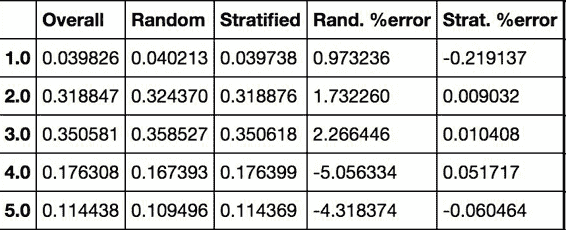for set in (strat_train_set, strat_test_set):
set.drop(["income_cat"], axis=1, inplace=True)


## 数据探索和可视化、发现规律

housing = strat_train_set.copy()


### 地理数据可视化

housing.plot(kind="scatter", x="longitude", y="latitude")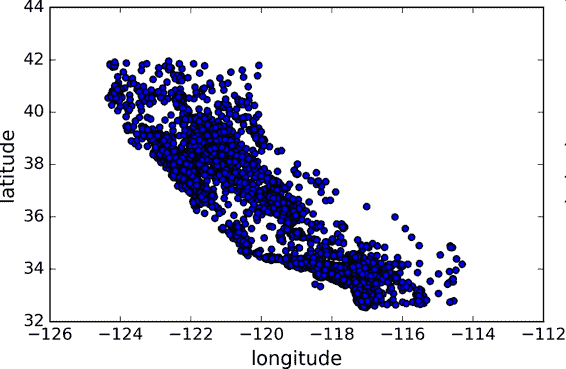housing.plot(kind="scatter", x="longitude", y="latitude", alpha=0.1)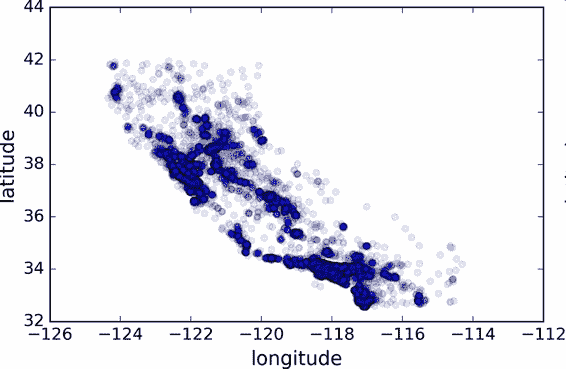housing.plot(kind="scatter", x="longitude", y="latitude", alpha=0.4,
s=housing["population"]/100, label="population",
c="median_house_value", cmap=plt.get_cmap("jet"), colorbar=True,
)
plt.legend()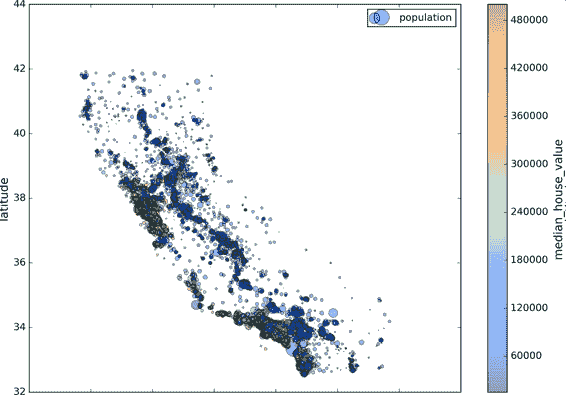### 查找关联

corr_matrix = housing.corr()


>>> corr_matrix["median_house_value"].sort_values(ascending=False)
median_house_value    1.000000
median_income         0.687170
total_rooms           0.135231
housing_median_age    0.114220
households            0.064702
total_bedrooms        0.047865
population           -0.026699
longitude            -0.047279
latitude             -0.142826
Name: median_house_value, dtype: float64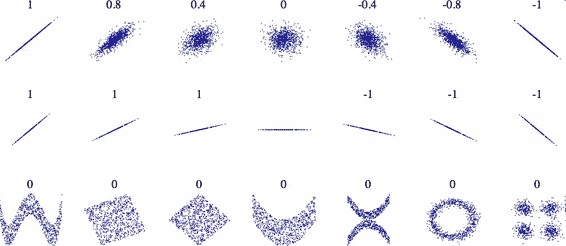from pandas.tools.plotting import scatter_matrix

attributes = ["median_house_value", "median_income", "total_rooms",
"housing_median_age"]
scatter_matrix(housing[attributes], figsize=(12, 8))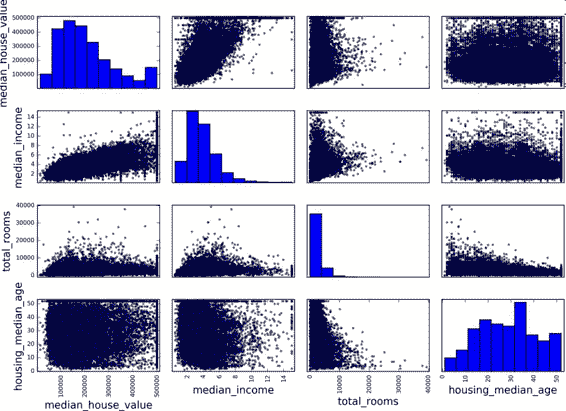housing.plot(kind="scatter", x="median_income",y="median_house_value",
alpha=0.1)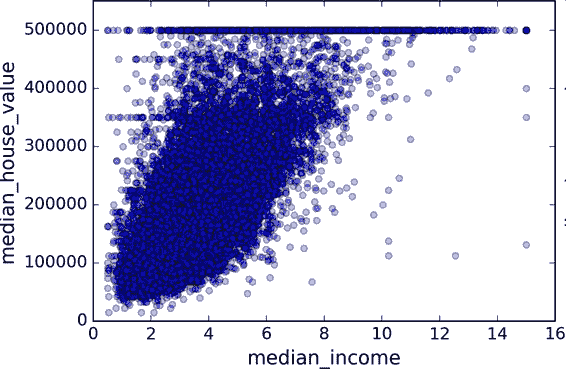### 属性组合试验

housing["rooms_per_household"] = housing["total_rooms"]/housing["households"]
housing["bedrooms_per_room"] = housing["total_bedrooms"]/housing["total_rooms"]
housing["population_per_household"]=housing["population"]/housing["households"]


>>> corr_matrix = housing.corr()
>>> corr_matrix["median_house_value"].sort_values(ascending=False)
median_house_value          1.000000
median_income               0.687170
rooms_per_household         0.199343
total_rooms                 0.135231
housing_median_age          0.114220
households                  0.064702
total_bedrooms              0.047865
population_per_household   -0.021984
population                 -0.026699
longitude                  -0.047279
latitude                   -0.142826
bedrooms_per_room          -0.260070
Name: median_house_value, dtype: float64


## 为机器学习算法准备数据

• 函数可以让你在任何数据集上（比如，你下一次获取的是一个新的数据集）方便地进行重复数据转换。

• 你能慢慢建立一个转换函数库，可以在未来的项目中复用。

• 在将数据传给算法之前，你可以在实时系统中使用这些函数。

• 这可以让你方便地尝试多种数据转换，查看哪些转换方法结合起来效果最好。

housing = strat_train_set.drop("median_house_value", axis=1)
housing_labels = strat_train_set["median_house_value"].copy()


### 数据清洗

• 去掉对应的街区；

• 去掉整个属性；

• 进行赋值（0、平均值、中位数等等）。

DataFramedropna()drop()，和fillna()方法，可以方便地实现：

housing.dropna(subset=["total_bedrooms"])    # 选项 1
housing.drop("total_bedrooms", axis=1)       # 选项 2
median = housing["total_bedrooms"].median()
housing["total_bedrooms"].fillna(median)     # 选项 3


Scikit-Learn 提供了一个方便的类来处理缺失值：Imputer。下面是其使用方法：首先，需要创建一个Imputer实例，指定用某属性的中位数来替换该属性所有的缺失值：

from sklearn.preprocessing import Imputer

imputer = Imputer(strategy="median")


housing_num = housing.drop("ocean_proximity", axis=1)


imputer.fit(housing_num)


imputer计算出了每个属性的中位数，并将结果保存在了实例变量statistics_中。虽然此时只有属性total_bedrooms存在缺失值，但我们不能确定在以后的新的数据中会不会有其他属性也存在缺失值，所以安全的做法是将imputer应用到每个数值：

>>> imputer.statistics_
array([ -118.51 , 34.26 , 29. , 2119. , 433. , 1164. , 408. , 3.5414])
>>> housing_num.median().values
array([ -118.51 , 34.26 , 29. , 2119. , 433. , 1164. , 408. , 3.5414])


X = imputer.transform(housing_num)


housing_tr = pd.DataFrame(X, columns=housing_num.columns)


Scikit-Learn 设计

Scikit-Learn 设计的 API 设计的非常好。它的主要设计原则是：

• 一致性：所有对象的接口一致且简单：

• 估计器（estimator）。任何可以基于数据集对一些参数进行估计的对象都被称为估计器（比如，imputer就是个估计器）。估计本身是通过fit()方法，只需要一个数据集作为参数（对于监督学习算法，需要两个数据集；第二个数据集包含标签）。任何其它用来指导估计过程的参数都被当做超参数（比如imputerstrategy），并且超参数要被设置成实例变量（通常通过构造器参数设置）。
• 转换器（transformer）。一些估计器（比如imputer）也可以转换数据集，这些估计器被称为转换器。API 也是相当简单：转换是通过transform()方法，被转换的数据集作为参数。返回的是经过转换的数据集。转换过程依赖学习到的参数，比如imputer的例子。所有的转换都有一个便捷的方法fit_transform()，等同于调用fit()transform()（但有时fit_transform()经过优化，运行的更快）。
• 预测器（predictor）。最后，一些估计器可以根据给出的数据集做预测，这些估计器称为预测器。例如，上一章的LinearRegression模型就是一个预测器：它根据一个国家的人均 GDP 预测生活满意度。预测器有一个predict()方法，可以用新实例的数据集做出相应的预测。预测器还有一个score()方法，可用于评估测试集（如果是监督学习算法的话，还要给出相应的标签）的预测质量。
• 可检验。所有估计器的超参数都可以通过实例的公共变量直接访问（比如，imputer.strategy），并且所有估计器学习到的参数也可以通过在实例变量名后加下划线来访问（比如，imputer.statistics_）。

• 类不可扩散。数据集被表示成 NumPy 数组或 SciPy 稀疏矩阵，而不是自制的类。超参数只是普通的 Python 字符串或数字。

• 可组合。尽可能使用现存的模块。例如，用任意的转换器序列加上一个估计器，就可以做成一个流水线，后面会看到例子。

• 合理的默认值。Scikit-Learn 给大多数参数提供了合理的默认值，很容易就能创建一个系统。

### 处理文本和类别属性

Scikit-Learn 为这个任务提供了一个转换器LabelEncoder

>>> from sklearn.preprocessing import LabelEncoder
>>> encoder = LabelEncoder()
>>> housing_cat = housing["ocean_proximity"]
>>> housing_cat_encoded = encoder.fit_transform(housing_cat)
>>> housing_cat_encoded
array([1, 1, 4, ..., 1, 0, 3])


py housing_cat_encoded, housing_categories = housing_cat.factorize() housing_cat_encoded[:10]

>>> print(encoder.classes_)
['<1H OCEAN' 'INLAND' 'ISLAND' 'NEAR BAY' 'NEAR OCEAN']


Scikit-Learn 提供了一个编码器OneHotEncoder，用于将整数分类值转变为独热向量。注意fit_transform()用于 2D 数组，而housing_cat_encoded是一个 1D 数组，所以需要将其变形：

>>> from sklearn.preprocessing import OneHotEncoder
>>> encoder = OneHotEncoder()
>>> housing_cat_1hot = encoder.fit_transform(housing_cat_encoded.reshape(-1,1))
>>> housing_cat_1hot
<16513x5 sparse matrix of type '<class 'numpy.float64'>'
with 16513 stored elements in Compressed Sparse Row format>


>>> housing_cat_1hot.toarray()
array([[ 0.,  1.,  0.,  0.,  0.],
[ 0.,  1.,  0.,  0.,  0.],
[ 0.,  0.,  0.,  0.,  1.],
...,
[ 0.,  1.,  0.,  0.,  0.],
[ 1.,  0.,  0.,  0.,  0.],
[ 0.,  0.,  0.,  1.,  0.]])


>>> from sklearn.preprocessing import LabelBinarizer
>>> encoder = LabelBinarizer()
>>> housing_cat_1hot = encoder.fit_transform(housing_cat)
>>> housing_cat_1hot
array([[0, 1, 0, 0, 0],
[0, 1, 0, 0, 0],
[0, 0, 0, 0, 1],
...,
[0, 1, 0, 0, 0],
[1, 0, 0, 0, 0],
[0, 0, 0, 1, 0]])


py

# Just run this cell, or copy it to your code, do not try to understand it (yet).

from sklearn.base import BaseEstimator, TransformerMixin from sklearn.utils import check_array from sklearn.preprocessing import LabelEncoder from scipy import sparse

class CategoricalEncoder(BaseEstimator, TransformerMixin): """Encode categorical features as a numeric array. The input to this transformer should be a matrix of integers or strings, denoting the values taken on by categorical (discrete) features. The features can be encoded using a one-hot aka one-of-K scheme (encoding='onehot', the default) or converted to ordinal integers (encoding='ordinal'). This encoding is needed for feeding categorical data to many scikit-learn estimators, notably linear models and SVMs with the standard kernels. Read more in the :ref:User Guide <preprocessing_categorical_features>. Parameters ---------- encoding : str, 'onehot', 'onehot-dense' or 'ordinal' The type of encoding to use (default is 'onehot'): - 'onehot': encode the features using a one-hot aka one-of-K scheme (or also called 'dummy' encoding). This creates a binary column for each category and returns a sparse matrix. - 'onehot-dense': the same as 'onehot' but returns a dense array instead of a sparse matrix. - 'ordinal': encode the features as ordinal integers. This results in a single column of integers (0 to n_categories - 1) per feature. categories : 'auto' or a list of lists/arrays of values. Categories (unique values) per feature: - 'auto' : Determine categories automatically from the training data. - list : categories[i] holds the categories expected in the ith column. The passed categories are sorted before encoding the data (used categories can be found in the categories_ attribute). dtype : number type, default np.float64 Desired dtype of output. handle_unknown : 'error' (default) or 'ignore' Whether to raise an error or ignore if a unknown categorical feature is present during transform (default is to raise). When this is parameter is set to 'ignore' and an unknown category is encountered during transform, the resulting one-hot encoded columns for this feature will be all zeros. Ignoring unknown categories is not supported for encoding='ordinal'. Attributes ---------- categories_ : list of arrays The categories of each feature determined during fitting. When categories were specified manually, this holds the sorted categories (in order corresponding with output of transform). Examples -------- Given a dataset with three features and two samples, we let the encoder find the maximum value per feature and transform the data to a binary one-hot encoding. >>> from sklearn.preprocessing import CategoricalEncoder >>> enc = CategoricalEncoder(handle_unknown='ignore') >>> enc.fit([[0, 0, 3], [1, 1, 0], [0, 2, 1], [1, 0, 2]]) ... # doctest: +ELLIPSIS CategoricalEncoder(categories='auto', dtype=<... 'numpy.float64'>, encoding='onehot', handle_unknown='ignore') >>> enc.transform([[0, 1, 1], [1, 0, 4]]).toarray() array([[ 1., 0., 0., 1., 0., 0., 1., 0., 0.], [ 0., 1., 1., 0., 0., 0., 0., 0., 0.]]) See also -------- sklearn.preprocessing.OneHotEncoder : performs a one-hot encoding of integer ordinal features. The OneHotEncoder assumes that input features take on values in the range [0, max(feature)] instead of using the unique values. sklearn.feature_extraction.DictVectorizer : performs a one-hot encoding of dictionary items (also handles string-valued features). sklearn.feature_extraction.FeatureHasher : performs an approximate one-hot encoding of dictionary items or strings. """

def __init__(self, encoding='onehot', categories='auto', dtype=np.float64,
handle_unknown='error'):
self.encoding = encoding
self.categories = categories
self.dtype = dtype
self.handle_unknown = handle_unknown

def fit(self, X, y=None):
"""Fit the CategoricalEncoder to X.
Parameters
----------
X : array-like, shape [n_samples, n_feature]
The data to determine the categories of each feature.
Returns
-------
self
"""

if self.encoding not in ['onehot', 'onehot-dense', 'ordinal']:
template = ("encoding should be either 'onehot', 'onehot-dense' "
"or 'ordinal', got %s")
raise ValueError(template % self.handle_unknown)

if self.handle_unknown not in ['error', 'ignore']:
template = ("handle_unknown should be either 'error' or "
"'ignore', got %s")
raise ValueError(template % self.handle_unknown)

if self.encoding == 'ordinal' and self.handle_unknown == 'ignore':
raise ValueError("handle_unknown='ignore' is not supported for"
" encoding='ordinal'")

X = check_array(X, dtype=np.object, accept_sparse='csc', copy=True)
n_samples, n_features = X.shape

self._label_encoders_ = [LabelEncoder() for _ in range(n_features)]

for i in range(n_features):
le = self._label_encoders_[i]
Xi = X[:, i]
if self.categories == 'auto':
le.fit(Xi)
else:
if self.handle_unknown == 'error':
msg = ("Found unknown categories {0} in column {1}"
" during fit".format(diff, i))
raise ValueError(msg)
le.classes_ = np.array(np.sort(self.categories[i]))

self.categories_ = [le.classes_ for le in self._label_encoders_]

return self

def transform(self, X):
"""Transform X using one-hot encoding.
Parameters
----------
X : array-like, shape [n_samples, n_features]
The data to encode.
Returns
-------
X_out : sparse matrix or a 2-d array
Transformed input.
"""
X = check_array(X, accept_sparse='csc', dtype=np.object, copy=True)
n_samples, n_features = X.shape
X_int = np.zeros_like(X, dtype=np.int)

for i in range(n_features):

if self.handle_unknown == 'error':
msg = ("Found unknown categories {0} in column {1}"
" during transform".format(diff, i))
raise ValueError(msg)
else:
# Set the problematic rows to an acceptable value and
# continue The rows are marked X_mask and will be
# removed later.
X_int[:, i] = self._label_encoders_[i].transform(X[:, i])

if self.encoding == 'ordinal':
return X_int.astype(self.dtype, copy=False)

n_values = [cats.shape for cats in self.categories_]
n_values = np.array( + n_values)
indices = np.cumsum(n_values)

row_indices = np.repeat(np.arange(n_samples, dtype=np.int32),

out = sparse.csc_matrix((data, (row_indices, column_indices)),
shape=(n_samples, indices[-1]),
dtype=self.dtype).tocsr()
if self.encoding == 'onehot-dense':
return out.toarray()
else:
return out




py

# from sklearn.preprocessing import CategoricalEncoder # in future versions of Scikit-Learn

cat_encoder = CategoricalEncoder() housing_cat_reshaped = housing_cat.values.reshape(-1, 1) housing_cat_1hot = cat_encoder.fit_transform(housing_cat_reshaped) housing_cat_1hot 

### 自定义转换器

from sklearn.base import BaseEstimator, TransformerMixin
rooms_ix, bedrooms_ix, population_ix, household_ix = 3, 4, 5, 6

def __init__(self, add_bedrooms_per_room = True): # no *args or **kargs
def fit(self, X, y=None):
return self  # nothing else to do
def transform(self, X, y=None):
rooms_per_household = X[:, rooms_ix] / X[:, household_ix]
population_per_household = X[:, population_ix] / X[:, household_ix]
bedrooms_per_room = X[:, bedrooms_ix] / X[:, rooms_ix]
return np.c_[X, rooms_per_household, population_per_household,
bedrooms_per_room]
else:
return np.c_[X, rooms_per_household, population_per_household]



### 转换流水线

from sklearn.pipeline import Pipeline
from sklearn.preprocessing import StandardScaler

num_pipeline = Pipeline([
('imputer', Imputer(strategy="median")),
('std_scaler', StandardScaler()),
])

housing_num_tr = num_pipeline.fit_transform(housing_num)


Pipeline构造器需要一个定义步骤顺序的名字/估计器对的列表。除了最后一个估计器，其余都要是转换器（即，它们都要有fit_transform()方法）。名字可以随意起。

from sklearn.base import BaseEstimator, TransformerMixin

class DataFrameSelector(BaseEstimator, TransformerMixin):
def __init__(self, attribute_names):
self.attribute_names = attribute_names
def fit(self, X, y=None):
return self
def transform(self, X):
return X[self.attribute_names].values


from sklearn.pipeline import FeatureUnion

num_attribs = list(housing_num)
cat_attribs = ["ocean_proximity"]

num_pipeline = Pipeline([
('selector', DataFrameSelector(num_attribs)),
('imputer', Imputer(strategy="median")),
('std_scaler', StandardScaler()),
])

cat_pipeline = Pipeline([
('selector', DataFrameSelector(cat_attribs)),
('label_binarizer', LabelBinarizer()),
])

full_pipeline = FeatureUnion(transformer_list=[
("num_pipeline", num_pipeline),
("cat_pipeline", cat_pipeline),
])


py cat_pipeline = Pipeline([ ('selector', DataFrameSelector(cat_attribs)), ('cat_encoder', CategoricalEncoder(encoding="onehot-dense")), ])

>>> housing_prepared = full_pipeline.fit_transform(housing)
>>> housing_prepared
array([[ 0.73225807, -0.67331551,  0.58426443, ...,  0.        ,
0.        ,  0.        ],
[-0.99102923,  1.63234656, -0.92655887, ...,  0.        ,
0.        ,  0.        ],
[...]
>>> housing_prepared.shape
(16513, 17)


## 选择并训练模型

### 在训练集上训练和评估

from sklearn.linear_model import LinearRegression

lin_reg = LinearRegression()
lin_reg.fit(housing_prepared, housing_labels)


>>> some_data = housing.iloc[:5]
>>> some_labels = housing_labels.iloc[:5]
>>> some_data_prepared = full_pipeline.transform(some_data)
>>> print("Predictions:\t", lin_reg.predict(some_data_prepared))
Predictions:     [ 303104.   44800.  308928.  294208.  368704.]
>>> print("Labels:\t\t", list(some_labels))
Labels:         [359400.0, 69700.0, 302100.0, 301300.0, 351900.0]


>>> from sklearn.metrics import mean_squared_error
>>> housing_predictions = lin_reg.predict(housing_prepared)
>>> lin_mse = mean_squared_error(housing_labels, housing_predictions)
>>> lin_rmse = np.sqrt(lin_mse)
>>> lin_rmse
68628.413493824875


OK，有总比没有强，但显然结果并不好：大多数街区的median_housing_values位于 120000 到 265000 美元之间，因此预测误差 68628 美元不能让人满意。这是一个模型欠拟合训练数据的例子。当这种情况发生时，意味着特征没有提供足够多的信息来做出一个好的预测，或者模型并不强大。就像前一章看到的，修复欠拟合的主要方法是选择一个更强大的模型，给训练算法提供更好的特征，或去掉模型上的限制。这个模型还没有正则化，所以排除了最后一个选项。你可以尝试添加更多特征（比如，人口的对数值），但是首先让我们尝试一个更为复杂的模型，看看效果。

from sklearn.tree import DecisionTreeRegressor

tree_reg = DecisionTreeRegressor()
tree_reg.fit(housing_prepared, housing_labels)


>>> housing_predictions = tree_reg.predict(housing_prepared)
>>> tree_mse = mean_squared_error(housing_labels, housing_predictions)
>>> tree_rmse = np.sqrt(tree_mse)
>>> tree_rmse
0.0


### 使用交叉验证做更佳的评估

from sklearn.model_selection import cross_val_score
scores = cross_val_score(tree_reg, housing_prepared, housing_labels,
scoring="neg_mean_squared_error", cv=10)
tree_rmse_scores = np.sqrt(-scores)


>>> def display_scores(scores):
...     print("Scores:", scores)
...     print("Mean:", scores.mean())
...     print("Standard deviation:", scores.std())
...
>>> display_scores(tree_rmse_scores)
Scores: [ 74678.4916885   64766.2398337   69632.86942005  69166.67693232
71486.76507766  73321.65695983  71860.04741226  71086.32691692
76934.2726093   69060.93319262]
Mean: 71199.4280043
Standard deviation: 3202.70522793


>>> lin_scores = cross_val_score(lin_reg, housing_prepared, housing_labels,
...                              scoring="neg_mean_squared_error", cv=10)
...
>>> lin_rmse_scores = np.sqrt(-lin_scores)
>>> display_scores(lin_rmse_scores)
Scores: [ 70423.5893262   65804.84913139  66620.84314068  72510.11362141
66414.74423281  71958.89083606  67624.90198297  67825.36117664
72512.36533141  68028.11688067]
Mean: 68972.377566
Standard deviation: 2493.98819069


>>> from sklearn.ensemble import RandomForestRegressor
>>> forest_reg = RandomForestRegressor()
>>> forest_reg.fit(housing_prepared, housing_labels)
>>> [...]
>>> forest_rmse
22542.396440343684
>>> display_scores(forest_rmse_scores)
Scores: [ 53789.2879722   50256.19806622  52521.55342602  53237.44937943
52428.82176158  55854.61222549  52158.02291609  50093.66125649
53240.80406125  52761.50852822]
Mean: 52634.1919593
Standard deviation: 1576.20472269


py from sklearn.externals import joblib

joblib.dump(my_model, "my_model.pkl")

# 然后

my_model_loaded = joblib.load("my_model.pkl") 

## 模型微调

### 网格搜索

from sklearn.model_selection import GridSearchCV

param_grid = [
{'n_estimators': [3, 10, 30], 'max_features': [2, 4, 6, 8]},
{'bootstrap': [False], 'n_estimators': [3, 10], 'max_features': [2, 3, 4]},
]

forest_reg = RandomForestRegressor()

grid_search = GridSearchCV(forest_reg, param_grid, cv=5,
scoring='neg_mean_squared_error')

grid_search.fit(housing_prepared, housing_labels)


param_grid告诉 Scikit-Learn 首先评估所有的列在第一个dict中的n_estimatorsmax_features3 × 4 = 12种组合（不用担心这些超参数的含义，会在第 7 章中解释）。然后尝试第二个dict中超参数的2 × 3 = 6种组合，这次会将超参数bootstrap设为False而不是True（后者是该超参数的默认值）。

>>> grid_search.best_params_
{'max_features': 6, 'n_estimators': 30}


>>> grid_search.best_estimator_
RandomForestRegressor(bootstrap=True, criterion='mse', max_depth=None,
max_features=6, max_leaf_nodes=None, min_samples_leaf=1,
min_samples_split=2, min_weight_fraction_leaf=0.0,
n_estimators=30, n_jobs=1, oob_score=False, random_state=None,
verbose=0, warm_start=False)


>>> cvres = grid_search.cv_results_
... for mean_score, params in zip(cvres["mean_test_score"], cvres["params"]):
...     print(np.sqrt(-mean_score), params)
...
64912.0351358 {'max_features': 2, 'n_estimators': 3}
55535.2786524 {'max_features': 2, 'n_estimators': 10}
52940.2696165 {'max_features': 2, 'n_estimators': 30}
60384.0908354 {'max_features': 4, 'n_estimators': 3}
52709.9199934 {'max_features': 4, 'n_estimators': 10}
50503.5985321 {'max_features': 4, 'n_estimators': 30}
59058.1153485 {'max_features': 6, 'n_estimators': 3}
52172.0292957 {'max_features': 6, 'n_estimators': 10}
49958.9555932 {'max_features': 6, 'n_estimators': 30}
59122.260006 {'max_features': 8, 'n_estimators': 3}
52441.5896087 {'max_features': 8, 'n_estimators': 10}
50041.4899416 {'max_features': 8, 'n_estimators': 30}
62371.1221202 {'bootstrap': False, 'max_features': 2, 'n_estimators': 3}
54572.2557534 {'bootstrap': False, 'max_features': 2, 'n_estimators': 10}
59634.0533132 {'bootstrap': False, 'max_features': 3, 'n_estimators': 3}
52456.0883904 {'bootstrap': False, 'max_features': 3, 'n_estimators': 10}
58825.665239 {'bootstrap': False, 'max_features': 4, 'n_estimators': 3}
52012.9945396 {'bootstrap': False, 'max_features': 4, 'n_estimators': 10}


### 随机搜索

• 如果你让随机搜索运行，比如 1000 次，它会探索每个超参数的 1000 个不同的值（而不是像网格搜索那样，只搜索每个超参数的几个值）。

• 你可以方便地通过设定搜索次数，控制超参数搜索的计算量。

### 分析最佳模型和它们的误差

>>> feature_importances = grid_search.best_estimator_.feature_importances_
>>> feature_importances
array([  7.14156423e-02,   6.76139189e-02,   4.44260894e-02,
1.66308583e-02,   1.66076861e-02,   1.82402545e-02,
1.63458761e-02,   3.26497987e-01,   6.04365775e-02,
1.13055290e-01,   7.79324766e-02,   1.12166442e-02,
1.53344918e-01,   8.41308969e-05,   2.68483884e-03,
3.46681181e-03])


>>> extra_attribs = ["rooms_per_hhold", "pop_per_hhold", "bedrooms_per_room"]
>>> cat_one_hot_attribs = list(encoder.classes_)
>>> attributes = num_attribs + extra_attribs + cat_one_hot_attribs
>>> sorted(zip(feature_importances,attributes), reverse=True)
[(0.32649798665134971, 'median_income'),
(0.15334491760305854, 'INLAND'),
(0.11305529021187399, 'pop_per_hhold'),
(0.07793247662544775, 'bedrooms_per_room'),
(0.071415642259275158, 'longitude'),
(0.067613918945568688, 'latitude'),
(0.060436577499703222, 'rooms_per_hhold'),
(0.04442608939578685, 'housing_median_age'),
(0.018240254462909437, 'population'),
(0.01663085833886218, 'total_rooms'),
(0.016607686091288865, 'total_bedrooms'),
(0.016345876147580776, 'households'),
(0.011216644219017424, '<1H OCEAN'),
(0.0034668118081117387, 'NEAR OCEAN'),
(0.0026848388432755429, 'NEAR BAY'),
(8.4130896890070617e-05, 'ISLAND')]


### 用测试集评估系统

final_model = grid_search.best_estimator_

X_test = strat_test_set.drop("median_house_value", axis=1)
y_test = strat_test_set["median_house_value"].copy()

X_test_prepared = full_pipeline.transform(X_test)

final_predictions = final_model.predict(X_test_prepared)

final_mse = mean_squared_error(y_test, final_predictions)
final_rmse = np.sqrt(final_mse)   # => evaluates to 48,209.6


## 练习

1. 尝试一个支持向量机回归器（sklearn.svm.SVR），使用多个超参数，比如kernel="linear"（多个超参数C值）。现在不用担心这些超参数是什么含义。最佳的SVR预测表现如何？

2. 尝试用RandomizedSearchCV替换GridSearchCV

3. 尝试在准备流水线中添加一个只选择最重要属性的转换器。

4. 尝试创建一个单独的可以完成数据准备和最终预测的流水线。

5. 使用GridSearchCV`自动探索一些准备过程中的候选项。